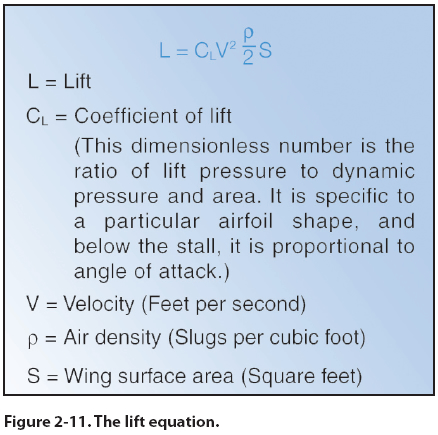Powered Parachute Flying Handbook Chapter 2 - Aerodynamics of Flight Lift Lift opposes the downward force of weight and is produced by the dynamic effects of the surrounding airstream acting on the wing. Lift acts perpendicular to the flight path through the wing’s center of lift. There is a mathematical relationship between lift, angle of attack, airspeed, altitude, and the size of the wing. In the lift equation, these factors correspond to the terms coefficient of lift, velocity, air density, and wing surface area. The relationship is expressed in Figure 2-11.This shows that for lift to increase, one or more of the factors on the other side of the equation must increase. Lift is proportional to the square of the velocity, or airspeed, therefore, doubling airspeed quadruples the amount of lift if everything else remains the same. Small changes in airspeed create larger changes in lift. Likewise, if other factors remain the same while the coefficient of lift increases, lift also will increase. The coefficient of lift goes up as the angle of attack is increased. As air density increases, lift increases. However, you will usually be more concerned with how lift is diminished by reductions in air density on a hot day, or if you are operating at higher altitudes. All wings produce lift in two ways: 1. Airfoil shape creating a higher velocity over the top of the wing and a lower velocity over the bottom of the wing with Bernoulli’s venturi effect. 2. Downward deflection of airflow because of the curvature of the wing with the principle of Newton’s Third Law of Motion: For every action, there is an equal and opposite reaction. Both principles determine the lifting force. Review Chapter 2 in the Pilot’s Handbook of Aeronautical Knowledge to understand Newton’s laws of motion and force and Bernoulli’s principle of pressure.
 ŠAvStop Online Magazine                                                                                                                                                       Contact Us              Return To Books